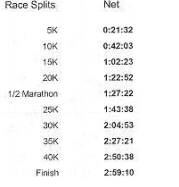# Running Kinematics Question

• physicsnobrain

## Homework Statement

A man decided to run a marathon.(a) What was his average speed, in m/s, for the first 5K?
(b) At 5K, was he speeding up or slowing down? Explain.

## Homework Equations

a) change in distance/time

b) acceleration = change in velocity/ change in time

## The Attempt at a Solution

So for part a I simply solve for average speed which is change in distance / time. I get 3.87m/s (I believe this is the right sig digs)

part b I'm not too sure if I am doing it right. I believe for him to be speeding up there needs to be acceleration @ 5K. So now I solve if there is acceleration at 5k. I start by doing acceleration = change in velocity / change in time.

So a = (3.87m/s - 0m/s) / (1292s - 0s)
a= 0.0039953 m/s^2

So the acceleration at 5k is 3.0x10^-3 m/s^2. And since there is some form of positive acceleration found at 5K, this means he is in fact speeding up at 5K.

Am I correct for part B, or completely wrong? I need help.

## Homework Statement

A man decided to run a marathon.(a) What was his average speed, in m/s, for the first 5K?
(b) At 5K, was he speeding up or slowing down? Explain.

## Homework Equations

a) change in distance/time

b) acceleration = change in velocity/ change in time

## The Attempt at a Solution

So for part a I simply solve for average speed which is change in distance / time. I get 3.87m/s (I believe this is the right sig digs)
'Looks right to me.So far so good.As far as the significant figures go however, you might want to hold onto a couple of more significant figures for later, since you will use this result in a future calculation. It may be okay to put "3.87 m/s" as the official answer for part (a), but privately hold onto more of them as you continue on to part (b).

part b I'm not too sure if I am doing it right. I believe for him to be speeding up there needs to be acceleration @ 5K. So now I solve if there is acceleration at 5k. I start by doing acceleration = change in velocity / change in time.

So a = (3.87m/s - 0m/s) / (1292s - 0s)
a= 0.0039953 m/s^2
I'm quite confident that's not the right approach. I don't think the question is asking you to find the runner's acceleration, certainly not during the first 5K anyway. I think it's safe to assume that the runner's acceleration is not uniform.

(Even if you were to assume that the runner had uniform acceleration for the first 5K-- which would lead to a really surreal situation btw, your approach still isn't right. The 3.87 m/s figure is the runner's average speed for the first 5K, not necessarily his final speed.)

So the acceleration at 5k is 3.0x10^-3 m/s^2. And since there is some form of positive acceleration found at 5K, this means he is in fact speeding up at 5K.

Am I correct for part B, or completely wrong? I need help.

You've found the average speed of the runner for the first 5K. What is the runner's speed during the second 5K? (I.e., what is the average speed between the 5K mark and the 10K mark?) Compare the two speeds (qualitatively compare them, that is).

'Looks right to me.So far so good.As far as the significant figures go however, you might want to hold onto a couple of more significant figures for later, since you will use this result in a future calculation. It may be okay to put "3.87 m/s" as the official answer for part (a), but privately hold onto more of them as you continue on to part (b).

I'm quite confident that's not the right approach. I don't think the question is asking you to find the runner's acceleration, certainly not during the first 5K anyway. I think it's safe to assume that the runner's acceleration is not uniform.

(Even if you were to assume that the runner had uniform acceleration for the first 5K-- which would lead to a really surreal situation btw, your approach still isn't right. The 3.87 m/s figure is the runner's average speed for the first 5K, not necessarily his final speed.)

You've found the average speed of the runner for the first 5K. What is the runner's speed during the second 5K? (I.e., what is the average speed between the 5K mark and the 10K mark?) Compare the two speeds (qualitatively compare them, that is).

OK. So I found the avg speed at 5K to be 3.86996m/s, and I found the avg speed at 10K to be 4.06173m/s. Therefore, since he has a faster average speed at 10K, does this mean he is speeding up at 5K?

Would this be an accurate and detailed answer?

OK. So I found the avg speed at 5K to be 3.86996m/s, and I found the avg speed at 10K to be 4.06173m/s. Therefore, since he has a faster average speed at 10K, does this mean he is speeding up at 5K?

Would this be an accurate and detailed answer?

Yes, I think you could argue that point.(And yes, by the way, ~4.06173 m/s is his average speed for the second 5K leg of the race. Good job.)

Yes, I think you could argue that point.(And yes, by the way, ~4.06173 m/s is his average speed for the second 5K leg of the race. Good job.)

Ok, thanks :D